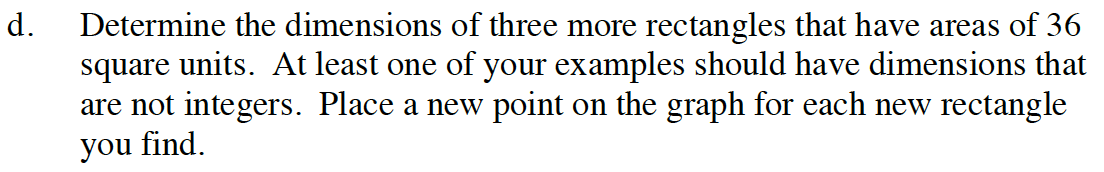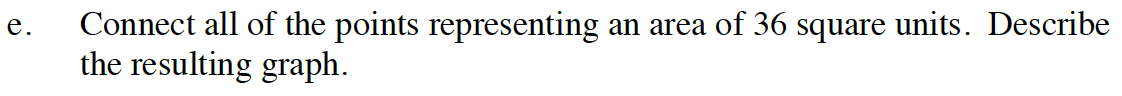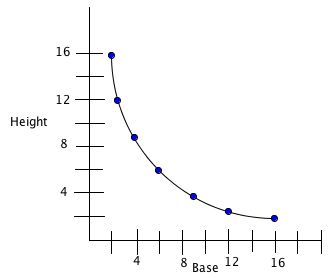Home > CC4 > Chapter A > Lesson A.1.7 > ProblemA-75

A-75.

Cairo wants to create a graph representing the heights and bases of all rectangles that have an area of 36 square units. He started by drawing the rectangles A, B, C, and D below. Examine the dimensions (length and width) of each rectangle. Homework Help ✎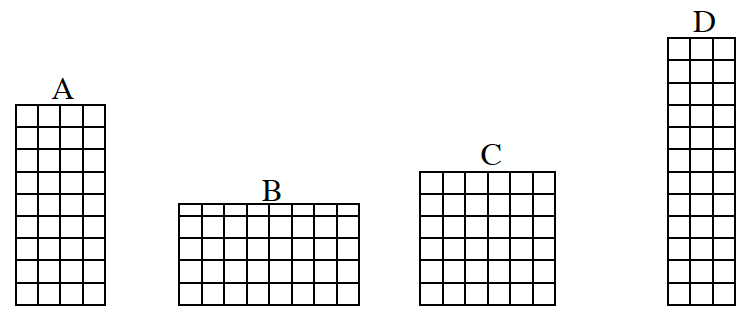1.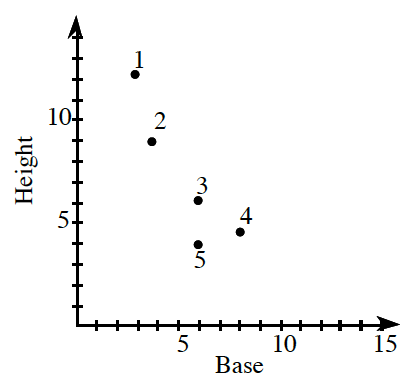Copy the graph at right onto graph paper. Then match the letter of each rectangle above with a point on the graph. Which point is not matched?

2. What are the base, height, and area for the unmatched point?

3. Why should the unmatched point not be on Cairo’s graph?

4. Determine the dimensions of three more rectangles that have areas of 36 square units. At least one of your examples should have dimensions that are not integers. Place a new point on the graph for each new rectangle you find.

5. Connect all of the points representing an area of 36 square units. Describe the resulting graph.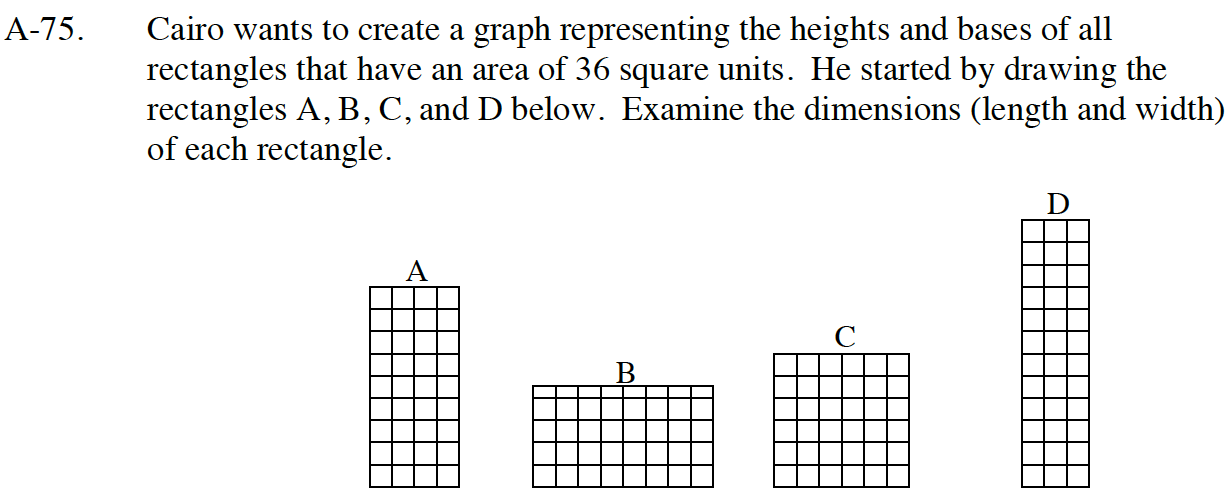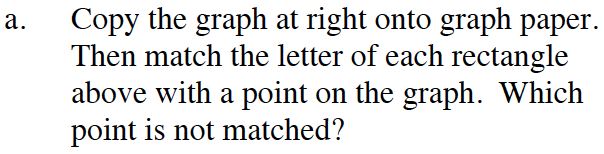Rectangle A has a height of 9 units and a baseof 4 units. Its corresponding point should be (4,9).

It corresponds to number 2.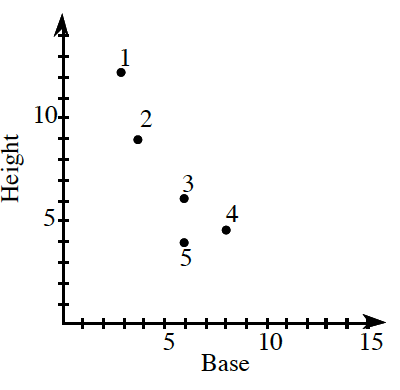Repeat for each rectangle.

You should find that 5 is not matched.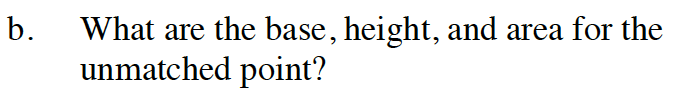Point 5 is 6 over and 4 up on the graph. Knowing this information, what are the rectangle's base, height, and area? Remember that the area is the base multiplied by the height.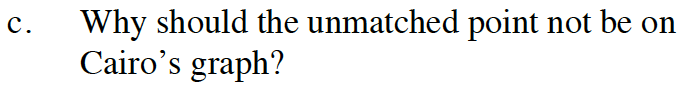Check the area of the unmatched rectangle that is representedby point 5.
Does that area meet the qualifications of Cairo's graph that only contains rectangles with areas that are equal to 36?